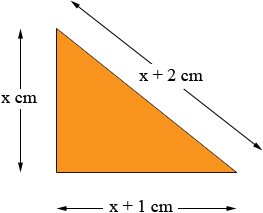SEARCH HOMEMath Central Quandaries & QueriesQuestion from bryan, a teacher: The sides of a right angled triangle are x, x+1, x+2 cm. Find the perimeter.?Bryan,

The lengths of the sides are x cm, x + 1 cm and x + 2 cm. Since this is a right angled triangle the hypotenuse is the longest side and hence its length must be x + 2 cm.Since this is a right angled triangle you can apply Pythagoras theorem and get

(x + 2)2 = x2 + (x + 1)2

Expand both sides and solve for x.

If you need further assistance write back.

PennyMath Central is supported by the University of Regina and The Pacific Institute for the Mathematical Sciences.